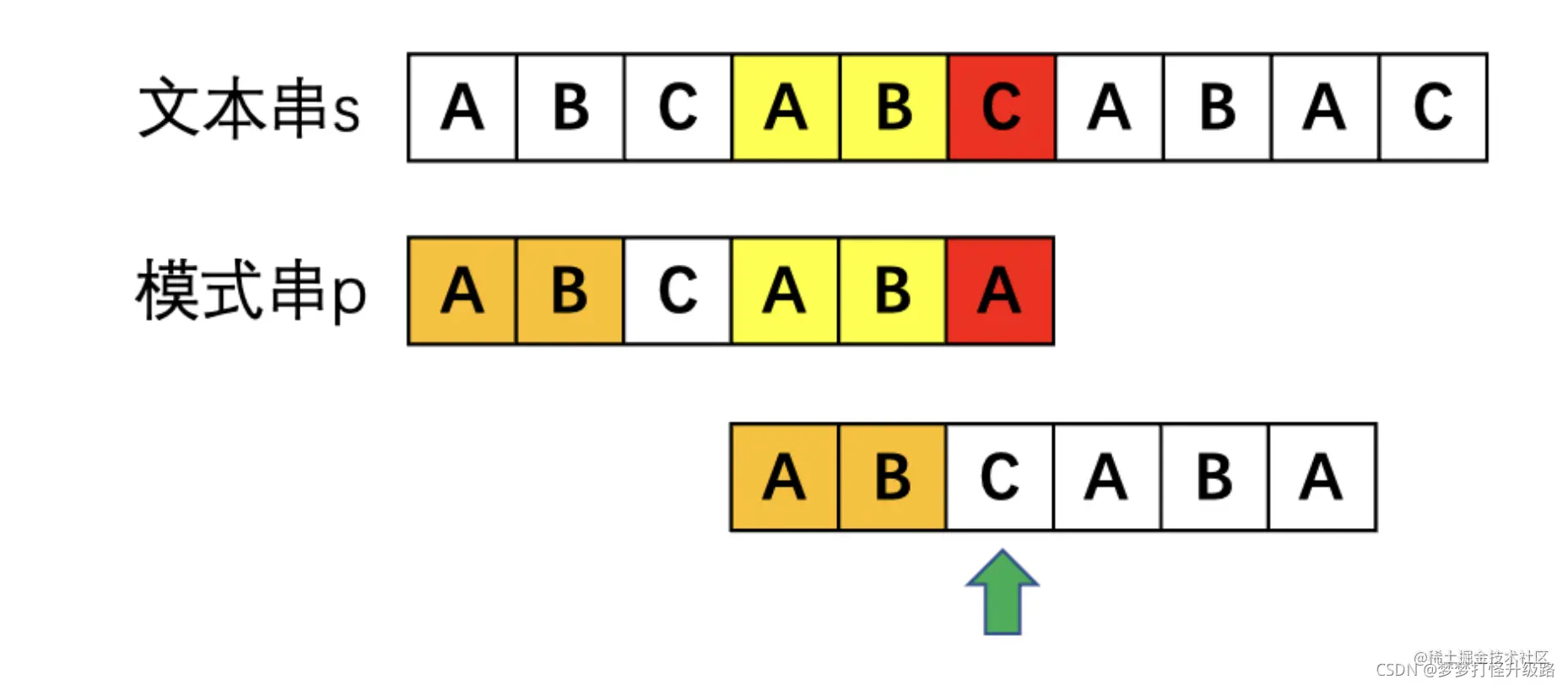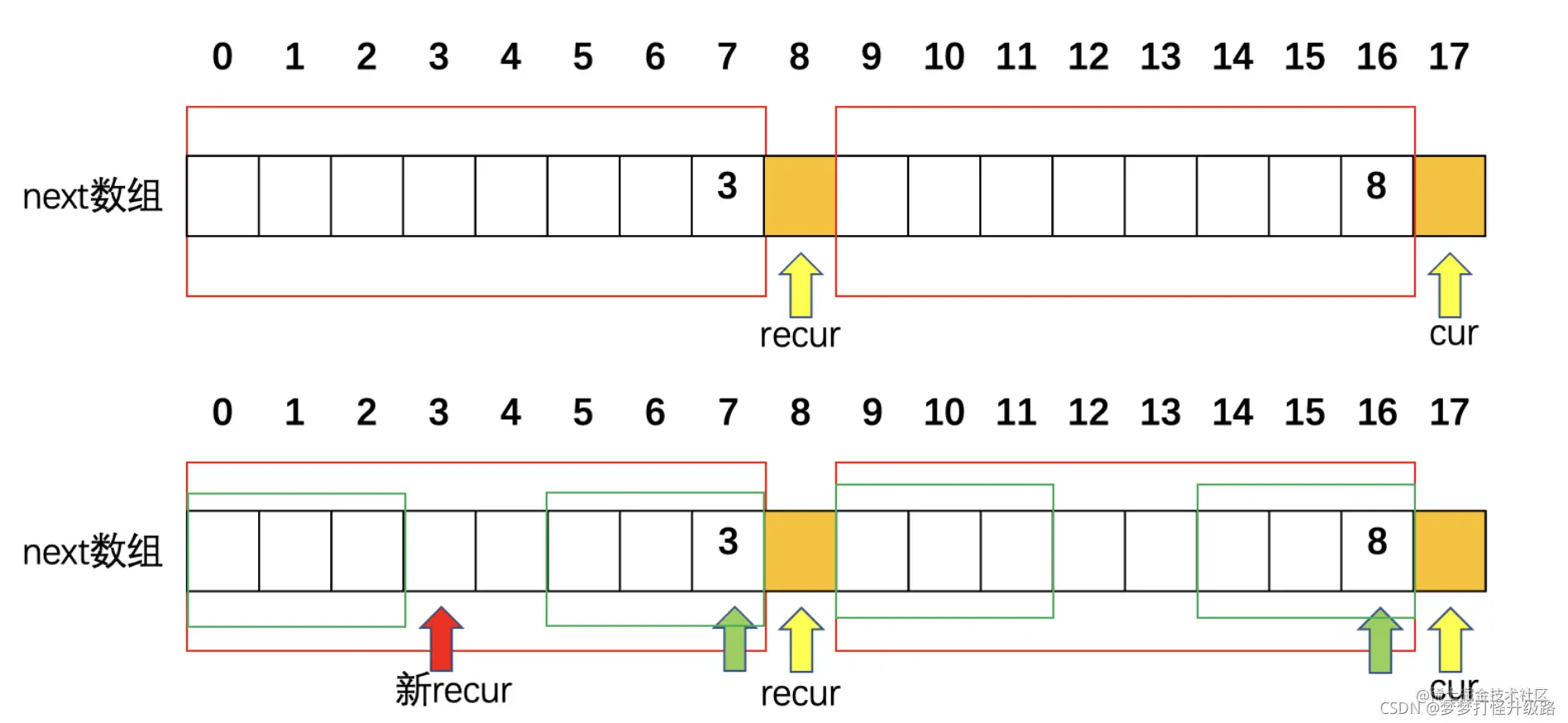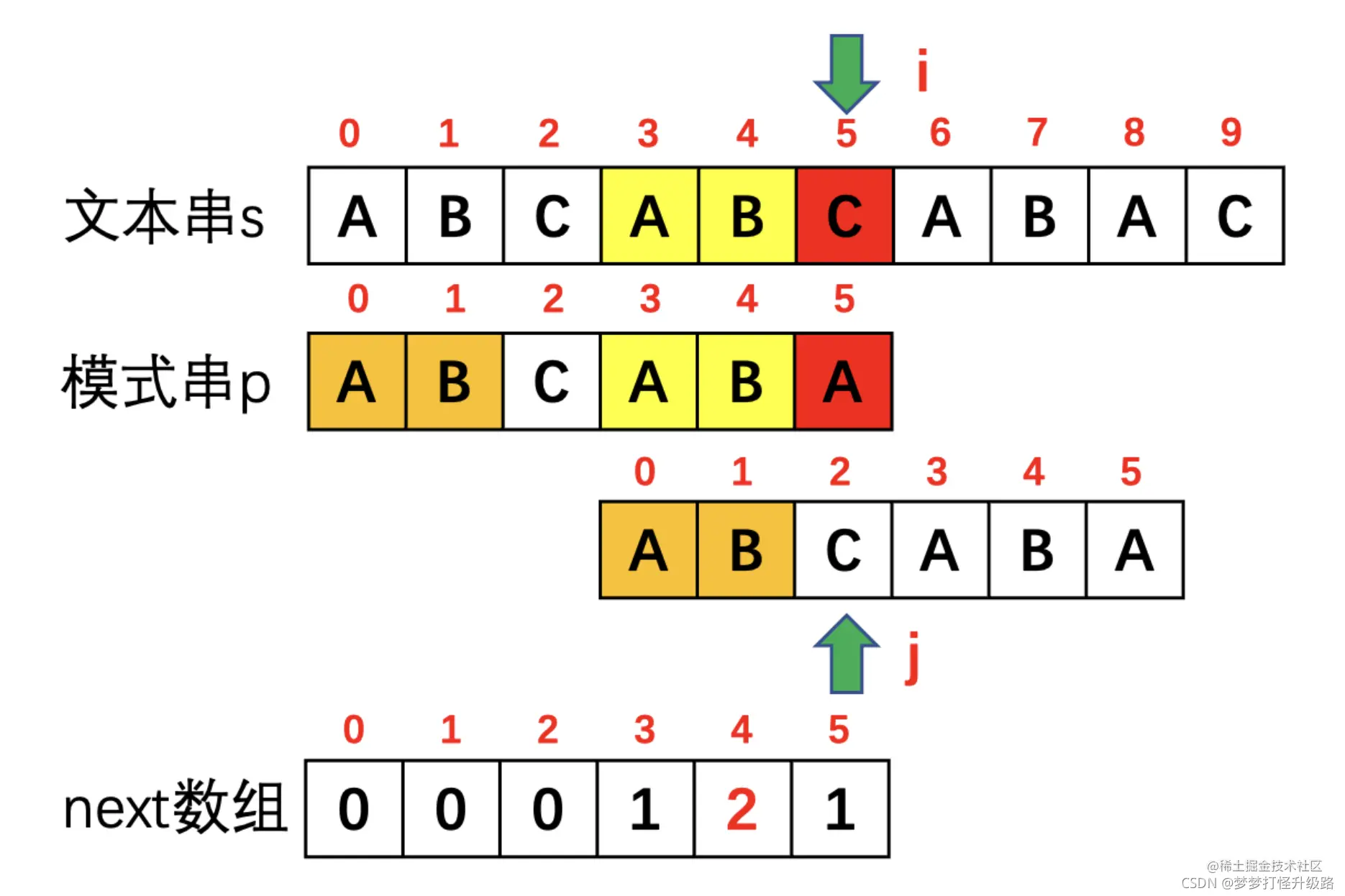# 零基础KMP算法讲清楚next数组生成代码KMP算法的由

KMP算法讲解的很多，清晰的很少，在此写一个汪汪都能看懂的通俗易懂直观的精讲。文末会给出学习建议。

# KMP算法的由来和本文术语解释

KMP算法的中文名称为快速模式匹配算法，是为了快速解决在一个字符串中查找另一个字符串的问题。（为了方便描述，要找到的那个字符串叫模式串，要搜索的那个范围叫文本串。比如要求在字符串abbaf中找到字符串baf，baf就是模式串，abbaf就是文本串）。

# 为什么要有next数组以及对他的直观理解

next数组就是一个模式串的行为指导手册。当红色的C和A不匹配时，黄色的AB和AB已经比较过了，而模式串p中橙色和黄色部分是相等的，那挺好，黄色AB都跟文本串比过了，橙色的AB就可以省点事，这样直接比较绿色箭头处就可以了。

# 精华！next数组的代码实现 图预警

## 递归思路如何画图理解

1.定义两个指针，cur是指向当前位置的，代表要求以cur位置字符结尾的最长相同前后缀，recur有两个含义，可能是上一轮循环之后传过来的（代表以前一个字符结尾的最长相同前后缀），也可能是递归的时候递归到的，反正也不用管这么多，反正recur在赋值给next时候就会指向最长相同前缀的下一位。

2.画图理解递归的思路next[cur-1]= next = 8代表红色的两个框相同（时刻牢记next的含义）

• 如果这个时候s[cur] == s[recur]说明next[cur] = recur+1=9,表示最长相同前后缀长度为9。
• 如果这个时候s[cur] != s[recur]说明next[cur] <= recur,这里就要用到递归了。红色框中字符相等，我们求的最长相同后缀是以cur结尾的那一撮，那就可以转化为求recur-1结尾的最长相同前后缀,即next[recur-1]。根据next[recur-1]=next的值3可以知道左边两个绿框相同，所以图中四个绿框就都相同了。但是咱要的其实还是左右边上的两个绿框相等。
• 接下来就还是循环第一步，recur指针移到新的红色位置。如果这时next[cur] == next[recur]的话，next[cur] = recur+1=4。如果不相等，那recur还要递归移动，直到recur = 0时不能再移动或者遇到相等的情况。
``````void getNext(int* next,string& s){
int recur = 0;
next = recur;
for(int cur = 1;cur < s.size();cur++){
while(recur > 0 && s[cur] != s[recur]){
recur = next[recur-1];//递归部分
}
if(s[cur] == s[recur]){//recur=0或者相等的时候
recur++;
}
next[cur] = recur;
}
}

# KMP算法的代码实现

## 利用next实现KMP的关键要点

1. 遇到某一位不匹配时，要根据这一位前一位的next值确认接下来要比较的是模式串中的哪一位(也就是模式串指针指向哪里)。
2. 1中改变了模式串指针之后，文本串指针不能移动到下一位，而是要保持不变，继续比较，否则就会越过一些值。所以for内部需要有while循环，而不能写if让其进入下一轮for循环。
``````  int j = 0;
for(int i = 0;i < haystack.size();i++){
while(j > 0 && needle[j] != haystack[i]){//对应要点2的while循环
cout << "pattern[" << j << "]" << "!=" << "s[" << i << "]" << endl;
cout << "j从" << j << "变为" << next[j-1] << endl;
j = next[j-1];//对应要点1的找前一位
}
if(needle[j] == haystack[i]){
cout << "pattern[" << j << "]" << "==" << "s[" << i << "]" << endl;
j++;
}
if(j == needle.size()) return i - needle.size()+1;//结束坐标-长度+1=起始坐标
}

## 运行结果的图形化解释

``````pattern==s
pattern==s
pattern==s
pattern==s
pattern==s
pattern!=s
j从5变为2
pattern==s
pattern==s
pattern==s
pattern==s